## What you need to know

• Students whose cumulative high school GPA is below 2.8 and who have not completed Algebra II in high school with a grade of “C-” or better should read through this guide to help determine which Math class is the best placement.
• Not all programs at LSC require college level math skills or a math course. You can refer to your program guide to see what skill level is needed to be successful in your program.
• Even if your program does not require a math course, LSC still requires that you to establish your math placement through the Guided Self Placement process, or through Accuplacer Placement Testing.
• If you are completing a transfer degree/AA/transfer pathway, college level math will be required. You can work with your advisor to determine which math class is best for your future educational goals.
• If this placement option is not the right fit, please schedule an Accuplacer placement test.

Thank you!

LSC Math Department and Advising Team

I am planning on taking higher math courses with my transfer program (such as College Algebra or Pre-Calculus).

Typically higher math courses are needed for Bachelor Degree programs in Math/Science/Computer Science and often Business/Management/Finance.

I am pursuing a technical program in Health, Business and Industry at Lake Superior College or I am planning on taking a non-math/science degree at a four-year institution.

## Are you ready for MATH 1100: College Algebra or MATH 1150: Pre-Calculus?

Topics you should be comfortable with before taking College Algebra or Pre-Calculus:

• Simplifying exponential expressions
• Operations of polynomials
• Factoring polynomials
• Operations on rational expressions
• Operations with complex numbers

Examples of skills needed for College Algebra:

1. Simplify the following exponential expressions:
1.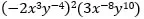2.2. Perform the following operations:
1.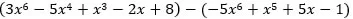2.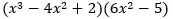3. Factor completely:
1.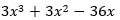2.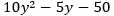3.4. Perform the following operations:
1.2.5. Simplify6. Solve the following equations:
1.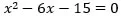(be able to solve by completing the square and the quadratic formula)
2.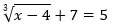7. Simplify and write using the imaginary unit8. Graph the function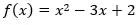## Are you ready for MATH 0970: Intermediate Algebra?

Topics you should be comfortable with prior to taking Intermediate Algebra:

• Rounding numbers
• Adding, subtracting, multiplying, dividing fractions, decimals and integers
• Converting between fractions, decimals, percentages
• Perform higher level Order of Operations
• Simplify one-variable equations
• Graphing and interpreting linear equations
• Interpret the slope of a linear equation
• Adding, subtracting, multiplying, dividing polynomial expressions
• Technical reading skills (interpret word problems)

Examples of skills needed for Intermediate Algebra:

1. Add without using a calculator and put in simplest form2. Writeas a decimal and percent (round to the nearest hundredth)
3. Evaluate the expression when x = 12 and y = 3,4. Solve: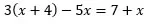5. Multiply: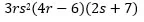6. Divide:7. Given the equationstate the slope, y-intercept, and graph the equation
8. The number of people N who have not been vaccinated is modeled by the equation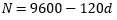where d is the number of days that vaccinations have been given. Find the number of people who have not been vaccinated after 16 days.

## Are you ready for MATH: 0955 or MATH 0950: Essential Skills in Mathematics?

MATH 0955 Essentials in Mathematics – Advanced

Topics you should be comfortable with before taking Essentials in Mathematics:

• Rounding numbers
• Adding, subtracting, multiplying, dividing fractions
• Adding, subtracting, multiplying, dividing decimals
• Adding, subtracting, multiplying, dividing integers (positive and negative)
• Converting between fractions, decimals, percentages
• Order of Operations

Examples of skills needed for Essentials in Mathematics without a calculator:

1. 418 + 289
2. 501 − 326
3. 13 × 79
4. 144 ÷ 6
5. Add and put in simplest form6. Multiple and put in simplest form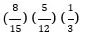7. 10 + (−1.2)
8. −23 − (−144)
9. (−2)(6)(−4)(−1)
10. 82 − 2(30 − 2 ∙ 4)

Math 0950 Essentials in Mathematics – Intermediate: If these skills are familiar to you, but you would benefit from additional support and skills refreshment in some areas, we recommend you take. This 5- credit class provides additional support and spends more time refreshing some of those basic skills.

*Non-credit courses are not eligible for Federal Financial Aid and do not count towards graduation – but there is no cost.

0955 and 0950 credits do not count towards your degree, but are eligible for Financial Aid funding and prepare you to take the math course required by your degree.

## What is ABE, Adult Education?

Learners will participate in whole class instruction while also working independently and/or in small groups on the math skills in their personally assessed area of need. The goal of Adult Education courses is to assist in transitioning students who want to build and/or refresh college level skills. Some basic computer skills are addressed.

ABE 0310 & ABE 0301 are free courses designed to prepare learners who are not yet ready for college ready math.

Lake Superior College Catalog

ABE 0310 – Pathways to College Success: Math Essentials I

This course is designed to help students to improve their math skills by developing the skills, habits, and attitudes that will result in accurate math analysis, attack and solution. Topics include numeracy strategies, basic arithmetic operations add/subtract, multiple and divide, fractions, decimals and precents, integers, graphing and algebra basics.

ABE 0301 – Pathways to College Success: Math Essentials II:

This course is designed to help students improve their math skills by developing the skills, habits, and attitudes that will result in accurate math analysis, attack and solution. Topics include numeracy strategies, basic arithmetic operations add/subtract, multiple and divide, fractions, decimals and precents, integers, graphing and algebra basics.

Learners will participate in whole class instruction while also working independently and/or in small groups on the math skills in their personally assessed area of need. The goal of Adult Education courses is to assist in transitioning students who want to build and/or refresh college level skills. Some basic computer skills are addressed.

These courses do not count towards a degree at LSC and they do not qualify for Financial Aid because there is no cost.

## Are you ready for MATH 1105: Mathematical Reasoning or MATH 1115: Contemporary Math?

Topics you should be comfortable with prior to taking Mathematical Reasoning or Contemporary Math:

• Rounding numbers
• Adding, subtracting, multiplying, dividing fractions, decimals and integers
• Converting between fractions, decimals, percentages
• Perform higher level Order of Operations
• Simplify and solve variable expressions and equations
• Computing exponential expressions and equations
• Graphing and interpreting linear equations
• Interpret the slope of a linear equation
• Interpret tables and descriptive graphs
• Technical reading skills (interpret word problems)

Examples of skills needed for Mathematical Reasoning:

1. Add without using a calculator and put in simplest form2. Writeas a decimal and percent (round to the nearest hundredth)
3. Evaluate the expression when x = 12 and 3 = 13,4. Given the equationstate the slope, y-intercept, and graph the equation
5. Simplify(a calculator may be used)
6. The number of people N who have not been vaccinated is modeled by the equation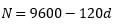where d is the number of days that vaccinations have been given. Find the number of people who have not been vaccinated after 16 days.
7. The bar graph shows the number of kilowatt hours of electricity generated by each fuel type. What percent of electricity is generated by Nuclear Energy?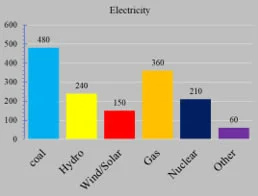## Are you ready for MATH 2210: General Statistics?

Topics you should be comfortable with before taking General Statistics:

• Rounding numbers
• Scientific notation and e-notation
• Adding, subtracting, multiplying, dividing fractions
• Converting between fractions, decimals, percentages
• Order of operations
• Evaluate variable expressions
• Computing sums using summation notation
• Graphing and interpreting linear equations
• Interpret the slope of a linear equation
• Technical reading skills (interpret word problems)

See below for some examples of skills needed for statistics:

1. Add without using a calculator and put in simplest form2. Write(that is in scientific notation) as a number
3. Writeas a decimal (round to the nearest hundredth) and a percent
4. Evaluatefor a = 4.3, b = 5.7, c = 2.3, d = 10
5. Given the equationstate the slope, y-intercept, and graph the equation
6. Given the table of values find (a) ∑ 𝑥2 and (b) ∑ 𝑥𝑦
 X -3 1 4 -5 3 Y 2 7 -2 01 -4
7. The number of people N who have not been vaccinated is modeled by the equation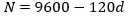where d is the number of days that vaccinations have been given. Find the number of people who have not been vaccinated after 16 days.

## Are you ready for MATH: 0955 or MATH 0950: Essential Skills in Mathematics?

MATH 0955 Essentials in Mathematics – Advanced

Topics you should be comfortable with before taking Essentials in Mathematics:

• Rounding numbers
• Adding, subtracting, multiplying, dividing fractions
• Adding, subtracting, multiplying, dividing decimals
• Adding, subtracting, multiplying, dividing integers (positive and negative)
• Converting between fractions, decimals, percentages
• Order of Operations

Examples of skills needed for Essentials in Mathematics without a calculator:

1. 418 + 289
2. 501 − 326
3. 13 × 79
4. 144 ÷ 6
5. Add and put in simplest form6. Multiply and put in simplest form7. 10 + (−1.2)
8. −23 − (−144)
9. (−2)(6)(−4)(−1)
10. 82 − 2(30 − 2 ∙ 4)

Math 0950 Essentials in Mathematics – Intermediate: If these skills are familiar to you, but you would benefit from additional support and skills refreshment in some areas, we recommend you take. This 5- credit class provides additional support and spends more time refreshing some of those basic skills.

*Non-credit courses are not eligible for Federal Financial Aid and do not count towards graduation – but there is no cost.

0955 and 0950 credits do not count towards your degree, but are eligible for Financial Aid funding and prepare you to take the math course required by your degree.

## What is ABE, Adult Education?

Learners will participate in whole class instruction while also working independently and/or in small groups on the math skills in their personally assessed area of need. The goal of Adult Education courses is to assist in transitioning students who want to build and/or refresh college level skills. Some basic computer skills are addressed.

ABE 0310 & ABE 0301 are free courses designed to prepare learners who are not yet ready for college ready math.

Lake Superior College Catalog

ABE 0310 – Pathways to College Success: Math Essentials I

This course is designed to help students to improve their math skills by developing the skills, habits, and attitudes that will result in accurate math analysis, attack and solution. Topics include numeracy strategies, basic arithmetic operations add/subtract, multiple and divide, fractions, decimals and precents, integers, graphing and algebra basics.

ABE 0301 – Pathways to College Success: Math Essentials II:

This course is designed to help students improve their math skills by developing the skills, habits, and attitudes that will result in accurate math analysis, attack and solution. Topics include numeracy strategies, basic arithmetic operations add/subtract, multiple and divide, fractions, decimals and precents, integers, graphing and algebra basics.